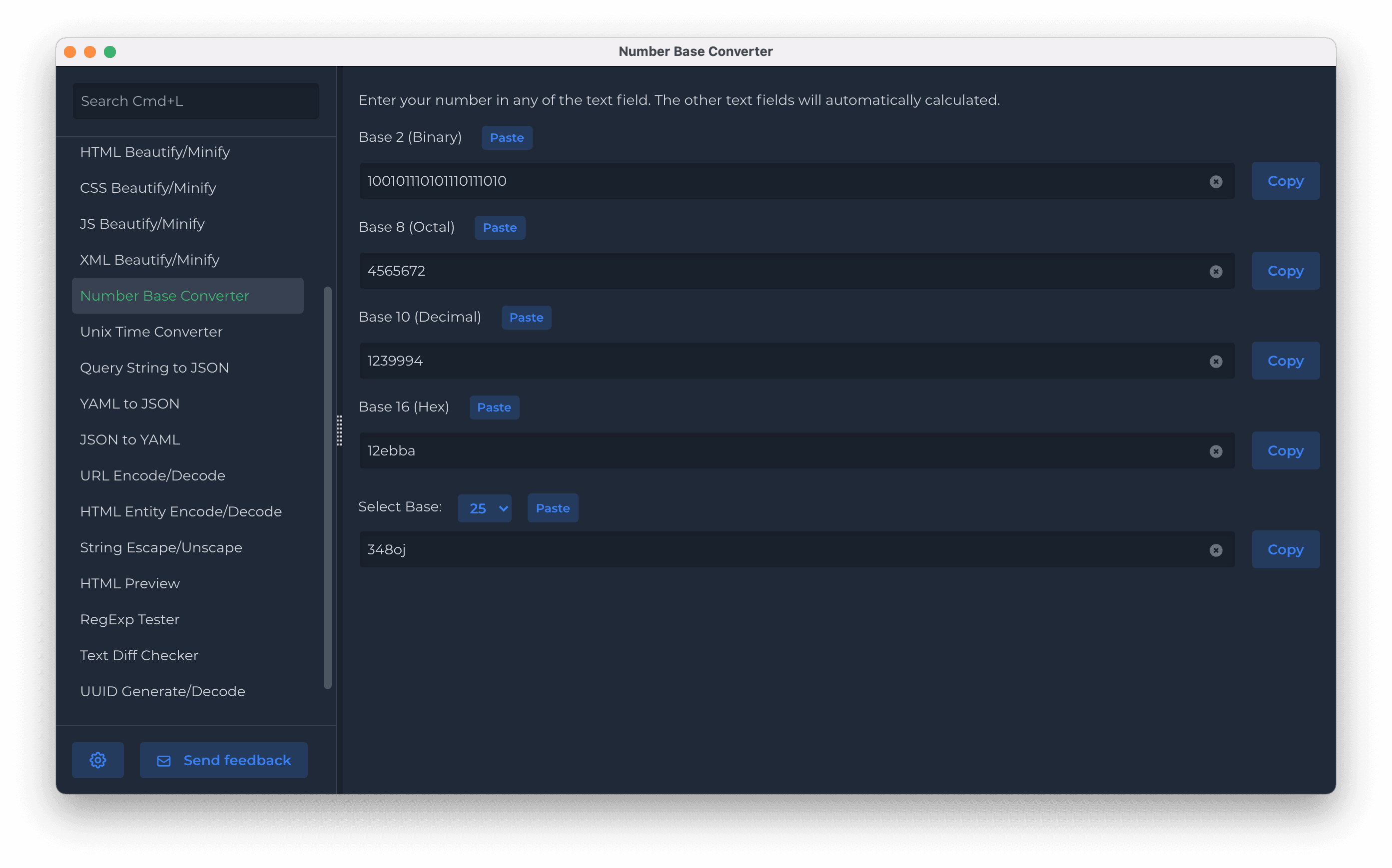# Number Base converter

This simple tool allows you to convert a same number between its representations under different number systems. For example, the number pi is equal to 3.14159... in a decimal system (base 10), or to 3.243F6... in a hexadecimal system (base 16), or to 11.001001... in a binary system (base 2).### Number Base

The most common number base is decimal, also known as base 10. The decimal number system uses 10 different notations which are the digits 0~9. Bases are not necessarily positive integers. Bases can be negative, positive, 0, complex and non-integral, too, although these are rarer. Other frequently used bases include base 2 and base 16. These are used in computing, and are called binary and hexadecimal, respectively.

### Number Base - Converting to Different Bases

As most commonly used number base system is decimal, we shall divide conversions into three parts:
• from any base to decimal number base
• from decimal to any number base
• from any base to any other base.
For now, we will learn about Integer number conversions and floating decimal conversions.
Wiki